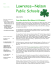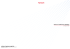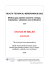# Section 11.9: The Gas Laws—Boyle`s Law, Gay Lussac`s Law, and

## Transcription

Section 11.9: The Gas Laws—Boyle`s Law, Gay Lussac`s Law, and
```Section 11.9: The Gas Laws—Boyle’s Law, Gay Lussac's Law, and the
Combined Gas Law
Mini Investigation: Modelling a Lung, page 555
A. Gas pressure is directly proportional to the number of collisions between gas entities and the
container. If the number of gas entities is constant and we expand the volume of the container,
there will be fewer collisions with the walls of the container. This means the pressure inside the
balloon will be reduced as the volume increases.
B. When we pull down on the balloon cover, the balloon inside inflates. The balloon cover acts
like the diaphragm in our chest cavity and the balloon inside the plastic cup acts like our lungs.
When we pull downward on the balloon, it creates a region of low pressure and outside air
moves into the balloon inside the cup. The air moves into the balloon because the air pressure
outside is greater than the pressure inside. This is similar to inhalation. In our chest cavity, our
diaphragm lowers creating low pressure in our lungs due to increased volume and air moves into
our lungs.
C. The model demonstrates inhalation showing the relationship between changes in volume and
inhalation but the demonstration does not show exhalation. The single balloon inside the cup
simulates the functioning two lungs. The details of lung anatomy are not shown.
Mini Investigation: Cartesian Diver, page 556
A. The eye dropper contains some water and a bubble of air. The density of the dropper with the
water and the air bubble is the same as the water in the pop bottle, so the diver initially floats.
B. As we apply pressure to the pop bottle we compress the air in the eye dropper. This causes the
air entities to compress, and more water moves into the diver. Water moving into the eyedropper
causes the eyedropper to become heavier and it sinks.
C. Submarines are composed of an inner and outer steel shell. In between is a region called the
hull. When floating on the surface, the hull is filled mostly with air. This is similar to the
Cartesian diver floating. To allow the submarine to dive the hull is filled with water, which
makes the submarine heavier and it sinks. The Cartesian diver sinks when more water is
introduced into the eyedropper by applying pressure.
Tutorial 1 Practice, page 559 1. Given: initial pressure, P1 = 446 kPa
initial volume, V1 = 8.25 L
final volume, V2 = 12 L
The amount of gas and the temperature remain constant.
Required: final pressure, P2
Analysis: Use Boyle’s law.
P1V1 = P2V2
Solution:
Step 1. Rearrange the equation to isolate the unknown variable.
PV
P2 = 1 1
V2
Chapter 11: The Gas State and Gas Laws
11.9-1
Step 2. Substitute given values (including units) into the equations and solve.
446 kPa ! 8.25 L
P2 =
12 L
P2 = 307 kPa
Statement: A pressure of 307 kPa must be applied to the gas when it occupies 12 L.
2. Given: final pressure, P2 = 2.84 kPa
initial volume, V1 = 1.75 L
final volume, V2 = 6.50 L
The amount of gas and the temperature remain constant.
Required: initial pressure, P1
Analysis: Use Boyle’s law.
P1V1 = P2V2
Solution:
Step 1. Rearrange the equation to isolate the unknown variable.
PV
P1 = 2 2
V1
Step 2. Substitute given values (including units) into the equations and solve.
2.84 kPa ! 6.50 L
P1 =
1.75 L
P1 = 10.5 kPa
Statement: The original pressure of the gas was 10.5 kPa.
3. Given: initial pressure, P1 = 85 kPa
initial temperature, t1 = 25 °C
final temperature, t2 = –11.4 °C
The volume and amount of gas remain constant.
Required: final pressure, P2
Analysis: Use Gay-Lussac's law.
P1 P2
=
T1 T2
Solution:
Step 1. Convert temperature values to kelvins.
T1 = t1 + 273
= 25 + 273
T1 = 298 K
T2 = t2 + 273
= –11.4 + 273
T2 = 261.6 K
Chapter 11: The Gas State and Gas Laws
11.9-2
Step 2. Rearrange the equation to isolate the unknown variable.
PT
P2 = 1 2
T1
Step 3. Solve the equation (including units).
85 kPa ! 261.6 K
P2 =
298 K
P2 = 75 kPa
Statement: The pressure of the gas inside the soccer ball is 75 kPa.
Tutorial 2 Practice, page 560 1. Given: initial pressure, P1 = 92.5 kPa
initial volume, V1 = 775 mL
initial temperature, t1 = –28 °C
final volume, V2 = 825 mL
final temperature, t2 = 15 °C
The amount of gas remains constant.
Required: final pressure, P2
Analysis: Use the combined gas law.
P1V1 P2V2
=
T1
T2
Solution:
Step 1. Convert temperature values to kelvins.
T1 = t1 + 273
= –28 + 273
T1 = 245 K
T2 = t2 + 273
= 15 + 273
T2 = 288 K
Step 2. Rearrange the equation to isolate the unknown variable.
PV T
P2 = 1 1 2
V2T1
Step 3. Substitute given values (including units) into the equation and solve.
92.5 kPa ! 775 mL ! 288 K
P2 =
825 mL ! 245 K
P2 = 102 kPa
Statement: The pressure at the bottom of the mountain is 102 kPa.
Chapter 11: The Gas State and Gas Laws
11.9-3
2. Given: initial pressure, P1 = 99.0 kPa
initial volume, V1 = 2.75 L
initial temperature, t1 = 21.0 °C
final pressure, P2 = 105 kPa
final temperature, t2 = 71.0 °C
Amount of gas remains constant.
Required: final volume, V2
Analysis: Use the combined gas law.
P1V1 P2V2
=
T1
T2
Solution:
Step 1. Convert temperature values to kelvins.
T1 = t1 + 273
= 21.0 + 273
T1 = 294 K
T2 = t2 + 273
= 71.0 + 273
T2 = 344 K
Step 2. Rearrange the equation to isolate the unknown variable.
PV T
V2 = 1 1 2
P2T1
Step 3. Substitute given values (including units) into the equation and solve.
99.0 kPa ! 2.75 L ! 344 K
V2 =
105 kPa ! 294 K
V2 = 3.03 L
Statement: The final volume of the gas is 3.03 L.
3. Given: initial pressure, P1 = 253 kPa
initial volume, V1 = 450 mL
initial temperature, t1 = 15 °C
final pressure, P2 = 405 kPa
final volume, V2 = 310 mL
Amount of gas remains constant.
Required: final temperature, t2
Analysis: Use the combined gas law.
P1V1 P2V2
=
T1
T2
Chapter 11: The Gas State and Gas Laws
11.9-4
Solution:
Step 1. Convert temperature values to kelvins.
T1 = t1 + 273
= 15 + 273
T1 = 288 K
Step 2. Rearrange the equation to isolate the unknown variable.
PV T
T2 = 2 2 1
P1V1
Step 3. Substitute given values (including units) into the equation and solve.
405 kPa ! 310 mL ! 288 K
T2 =
253 kPa ! 450 mL
T2 = 317.6 K [two extra digits carried]
Step 4. Convert temperature values back to Celsius.
T2 = t2 + 273
t2 = T2 ! 273
= 318 ! 273
t2 = 45 º C
Statement: The final temperature is 45 °C.
Research This: Investigating Gas Law Simulations, page 551
A. Three important criteria for the gas law simulations are ease-of-use, efficient display of data,
and accuracy of the data in describing the gas law. These criteria were chosen because these
reflect some of the advantages of using a computer simulation over a textbook or other resource.
B. I chose the “Gas Properties” simulation hosted by the University of Colorado at Boulder
because it simulated a wide range of gas phenomena as well as scoring the highest out of all the
simulations for our three chosen criteria.
C. I would improve the simulations’ instructions to make them clearer, more easily found, and
have more information. I would also improve the visuals of the simulation.
D. Using simulations helps to visualize how different quantities are related and how they change
with time. Using a simulation allows you to see how changing one variable affects other
variables instantly, and having a corresponding visual aid (such as molecules being pumped into
a box representing the pressure variable) makes it easier to understand why the affected variables
change in the way they do.
Chapter 11: The Gas State and Gas Laws
11.9-5
Section 11.9 Questions, page 562
1.
2. Given: initial volume, V1 = 45.2 L
initial temperature, T1 = 280 K
final temperature, T2 = 560 K
The initial pressure is equal to final pressure.
The amount of gas remains constant.
Required: final volume, V2
Analysis: Use Charles’ law. Use
V1 V2
=
T1 T2
to simplify the equation.
V1 V2
=
T1 T2
Solution:
Step 1. Rearrange the equation to isolate the unknown variable.
VT
V2 = 1 2
T1
Step 2. Substitute given values (including units) into the equation and solve.
45.2 L ! 560 K
V2 =
280 K
V2 = 9.0 ! 10 L
Statement: The final volume of the nitrogen gas is 9.0 × 10 L.
Chapter 11: The Gas State and Gas Laws
11.9-6
3. Given: initial pressure, P1 = 340 kPa
initial temperature, t1 = 15 °C
final temperature, t2 = –15 °C
The volume and amount of gas remain constant.
Required: final pressure, P2
Analysis: Use Gay-Lussac’s law.
P1 P2
=
T1 T2
Solution:
Step 1. Convert temperature values to kelvins.
T1 = t1 + 273
= 15 + 273
T1 = 288 K
T2 = t2 + 273
= –15 + 273
T2 = 258 K
Step 2. Rearrange the equation to isolate the unknown variable.
PT
P2 = 1 2
T1
Step 3. Solve the equation (including units).
340 kPa ! 258 K
P2 =
288 K
P2 = 3.0 ! 102 kPa
Statement: The new pressure will be 3.0 × 102 kPa.
4. (a) Given: initial pressure, P1 = 101.3 kPa
initial volume, V1 = 3.50 L
initial temperature, T1 = 320 K
final volume, V2 = 8.40 L
final temperature, T2 = 320 K
The amount of gas and the temperature remain constant.
Required: final pressure, P2
Analysis: Use Boyle’s law.
P1V1 = P2V2
Solution:
Step 1. Rearrange the equation to isolate the unknown variable.
PV
P2 = 1 1
V2
Chapter 11: The Gas State and Gas Laws
11.9-7
Step 2. Substitute given values (including units) into the equations and solve.
101.3 kPa ! 3.50 L
P2 =
8.40 L
P2 = 42.2 kPa
Statement: The final pressure is 42.2 kPa.
(b) Given: initial pressure, P1 = 210 kPa
initial temperature, T1 = 415 K
final pressure, P2 = 420 kPa
final volume, V2 = 120 mL
final temperature, T2 = 415 K
The amount of gas and the temperature remain constant.
Required: initial volume, V1
Analysis: Use Boyle’s law.
P1V1 = P2V2
Solution:
Step 1. Rearrange the equation to isolate the unknown variable.
PV
V1 = 2 2
P1
Step 2. Substitute given values (including units) into the equations and solve.
420 kPa ! 120 mL
V1 =
210 kPa
V1 = 240 mL
Statement: The initial volume is 240 mL.
(c) Given: initial pressure, P1 = 720 mm Hg
initial volume, V1 = 345 mL
initial temperature, T1 = 420 K
final pressure, P2 = 620 mm Hg
final temperature, T2 = 640 K
The amount of gas remains constant.
Required: final volume, V2
Analysis: Use the combined gas law.
P1V1 P2V2
=
T1
T2
Solution:
Step 1. Rearrange the equation to isolate the unknown variable.
PV T
V2 = 1 1 2
P2T1
Chapter 11: The Gas State and Gas Laws
11.9-8
Step 2. Substitute given values (including units) into the equation and solve.
720 mm Hg ! 345 mL ! 640 K
V2 =
620 mm Hg ! 420 K
V2 = 610 mL
Statement: The final volume, V2, is 610 mL.
5. Given: initial pressure, P1 = 130 kPa
initial temperature, t1 = 6.50 °C
final temperature, t2 = 670.0 °C
The volume and amount of gas remain constant.
Required: final pressure, P2
Analysis: Use Gay-Lussac’s law.
P1 P2
=
T1 T2
Solution:
Step 1. Convert temperature values to kelvins.
T1 = t1 + 273
= 6.50 + 273
T1 = 279.50 K
T2 = t2 + 273
= 670.0 + 273
T2 = 943.0 K
Step 2. Rearrange the equation to isolate the unknown variable.
PT
P2 = 1 2
T1
Step 3. Solve the equation (including units).
130 kPa ! 943.0 K
P2 =
279.50 K
P2 = 4.4 ! 102 kPa
Statement: The new pressure of the neon gas is 4.4 × 102 kPa.
6. Given: initial pressure, P1 = 95 kPa
initial temperature, t1 = 22 °C
final pressure, P2 = 350 kPa
The volume and amount of gas remain constant.
Required: final temperature, t2
Analysis: Use Gay-Lussac’s law.
P1 P2
=
T1 T2
Chapter 11: The Gas State and Gas Laws
11.9-9
Solution:
Step 1. Convert temperature values to kelvins.
T1 = t1 + 273
= 22 + 273
T1 = 295 K
Step 2. Rearrange the equation to isolate the unknown variable.
PT
T1 = 1 2
P2
Step 3. Solve the equation (including units).
T2 =
350 kPa ! 295 K
95 kPa
T2 = 1.087 ! 103 K
Step 4. Convert temperatures values back to Celsius.
T2 = t2 + 273
t2 = T2 ! 273
= 1.087 " 103 ! 273
t2 = 8.1 " 102 °C
Statement: The tank can sustain a maximum temperature of 8.1 × 102 °C.
7. Given: initial pressure, P1 = 101.3 kPa
initial temperature, t1 = 20 °C
final temperature, t2 = –15 °C
The volume and amount of gas remain constant.
Required: final pressure, P2
Analysis: Use Gay-Lussac’s law.
P1 P2
=
T1 T2
Solution:
Step 1. Convert temperature values to kelvins.
T1 = t1 + 273
= 20 + 273
T1 = 293 K
T2 = t2 + 273
= –15 + 273
T2 = 258 K
Step 2. Rearrange the equation to isolate the unknown variable.
PT
P2 = 1 2
T1
Chapter 11: The Gas State and Gas Laws
11.9-10
Step 3. Solve the equation (including units).
101.3 kPa ! 258 K
P2 =
293 K
P2 = 89.2 kPa
Statement: The final pressure of the nitrogen gas is 89.2 kPa.
8. Given: initial pressure, P1 = 152 kPa
final temperature, t2 = 200 °C
The initial temperature, t1, is standard ambient temperature, which is 25 °C.
The volume and amount of gas remain constant.
Required: final pressure, P2
Analysis: Use Gay-Lussac’s law.
P1 P2
=
T1 T2
Solution:
Step 1. Convert temperature values to kelvins.
T1 = t1 + 273
= 25 + 273
T1 = 298 K
T2 = t2 + 273
= 200 + 273
T2 = 473 K
Step 2. Rearrange the equation to isolate the unknown variable.
PT
P2 = 1 2
T1
Step 3. Solve the equation (including units) to determine if the final pressure is greater than 253
kPa.
152 kPa ! 473 K
P2 =
298 K
P2 = 241 kPa
Statement: The pressure of the gas at 200 °C is 241 kPa. This is not greater than the 253 kPa
limit, so yes, the glassware can withstand the gas being heated to this temperature.
9. (a) I would expect the tire pressure to decrease. As the temperature decreases the motion of
entities will decrease. This will reduce the volume the entities occupy and reduce the number of
collisions with the tire surface, thereby reducing the pressure.
(b) Given: initial pressure, P1 = 227.5 kPa
initial temperature, t1 = 10 °C
final temperature, t2 = –5 °C
The volume and amount of gas remain constant.
Required: final pressure, P2
Chapter 11: The Gas State and Gas Laws
11.9-11
Analysis: Use Gay-Lussac's law.
P1 P2
=
T1 T2
Solution:
Step 1. Convert temperature values to kelvins.
T1 = t1 + 273
= 10 + 273
T1 = 283 K
T2 = t2 + 273
= –5 + 273
T2 = 268 K
Step 2. Rearrange the equation to isolate the unknown variable.
PT
P2 = 1 2
T1
Step 3. Solve the equation (including units).
227.5 kPa ! 268 K
P2 =
283 K
P2 = 2.2 ! 102 kPa
Statement: The new tire pressure is 2.2 × 102 kPa.
10. Given: initial pressure, P1 = 125 kPa
initial volume, V1 = 1250 cm3
final pressure, P2 = 97 kPa
The amount of gas and the temperature remain constant.
Required: final volume, V2
Analysis: Use Boyle’s law.
P1V1 = P2V2
Solution:
Step 1. Rearrange the equation to isolate the unknown variable.
PV
V2 = 1 1
P2
Step 2. Substitute given values (including units) into the equations and solve.
V2 =
125 kPa ! 1250 cm 3
97 kPa
V2 = 1.6 ! 103 cm 3
Statement: The volume of the balloon at the new altitude would be 1.6 × 103 cm3.
Chapter 11: The Gas State and Gas Laws
11.9-12
11. Given: initial pressure, P1 = 2.7574 × 104 kPa
initial volume, V1 = 4.50 × 104 L
final volume, V2 = 6.00 × 104 L
The amount of gas and the temperature remain constant.
Required: final pressure, P2
Analysis: Use Boyle’s law.
P1V1 = P2V2
Solution:
Step 1. Rearrange the equation to isolate the unknown variable.
PV
P2 = 1 1
V2
Step 2. Substitute given values (including units) into the equations and solve.
2.7574 ! 104 kPa ! 4.50 ! 104 L
P2 =
6.00 ! 104 L
P2 = 2.07 ! 104 kPa
Statement: The new pressure would be 2.07 × 104 kPa.
12. (a) Answers may vary. Some examples of gases stored under pressure are: oxygen for
patients, helium for balloons, and acetylene torches for welding.
(b) These gases need to be stored safely and away from incompatible materials. The cylinders
must be well maintained and container seals must be in good condition. The containers must be
transported carefully.
(c) These gases need to be stored away from incompatible materials, since they could react if any
gas leaks. They need to be stored safely to minimize the risk of fires, spills, or leaks. In the case
of fire, as the temperature increases, the gases would expand and the pressure inside the cylinder
would increase. If the pressure inside the storage tanks exceeds the limits of the cylinder an
explosion could occur. They should be stored away from busy areas. If the tanks are damaged
they could leak or explode.
The gas cylinders must be checked regularly to ensure that all valves are working
properly. The cylinders must be inspected for leaks or any dents that could release gas. Gases
that escape could react with entities in the environment.
These Gases must be transported carefully so the containers are not damaged, otherwise
release of gas or a container explosion could occur.
13. Given: initial pressure, P1 = 685 kPa
initial volume, V1 = 8.0 mL
initial temperature, t1 = 12 °C
final pressure, P2 = 99 kPa
final temperature, t2 = 26 °C
The amount of gas remains constant.
Required: final volume, V2
Analysis: Use the combined gas law.
P1V1 P2V2
=
T1
T2
Chapter 11: The Gas State and Gas Laws
11.9-13
Solution:
Step 1. Convert temperature values to kelvins.
T1 = t1 + 273
= 12 + 273
T1 = 285 K
T2 = t2 + 273
= 26 + 273
T2 = 299 K
Step 2. Rearrange the equation to isolate the unknown variable.
PV T
V2 = 1 1 2
P2T1
Step 3. Substitute given values (including units) into the equation and solve.
685 kPa ! 8.0 mL ! 299 K
V2 =
99 kPa ! 285 K
V2 = 58 mL
Statement: The volume of the gas bubble when it reaches the surface is 58 mL.
14. Given: initial pressure, P1 = 112 kPa
initial volume, V1 = 5.50 L
initial temperature, t1 = 25 °C
final pressure, P2 = 32.0 kPa
final temperature, t2 = –34 °C
The amount of gas remains constant.
Required: final volume, V2
Analysis: Use the combined gas law.
P1V1 P2V2
=
T1
T2
Solution:
Step 1. Convert temperature values to kelvins.
T1 = t1 + 273
= 25 + 273
T1 = 298 K
T2 = t2 + 273
= !34 + 273
T2 = 239 K
Step 2. Rearrange the equation to isolate the unknown variable.
PV T
V2 = 1 1 2
P2T1
Chapter 11: The Gas State and Gas Laws
11.9-14
Step 3. Substitute given values (including units) into the equation and solve.
112 kPa ! 5.50 L ! 239 K
V2 =
32.0 kPa ! 298 K
V2 = 15.4 L
Statement: The final volume of the balloon is 15.4 L.
15. Given: initial pressure, P1 = 116 kPa
initial volume, V1 = 893 mL
initial temperature, t1 = 44 °C
final pressure, P2 = 102 kPa
final volume, V2 = 1.03 L
The amount of gas remains constant.
Required: final temperature, t2
Analysis: Use the combined gas law.
P1V1 P2V2
=
T1
T2
Solution:
Step 1. Convert temperature values to kelvins and volume values to litres.
T1 = t1 + 273
= 44 + 273
T1 = 317 K
V2 = 893 mL
= 893 mL !
1L
1000 mL
V2 = 0.893 L
Step 2. Rearrange the equation to isolate the unknown variable.
PV T
T2 = 2 2 1
P1V1
Step 3. Substitute given values (including units) into the equation and solve.
102 kPa ! 1.03 L ! 317 K
T2 =
116 kPa ! 0.893 L
T2 = 321.5 K [two extra digits carried]
Chapter 11: The Gas State and Gas Laws
11.9-15
Step 4. Convert temperature values back to Celsius.
T2 = t2 + 273
t2 = T2 ! 273
= 321.5 ! 273
t2 = 49 º C
Statement: The temperature at which the gas will occupy a volume of 1.03 L at a pressure of
102 kPa is 49 °C.
Chapter 11: The Gas State and Gas Laws
11.9-16
```

### CATALOG - Maxitrol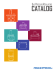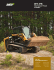### Gas Pressure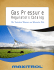### Alfa Laval plate heat exchangers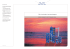### guidelines for voluntary water efficient products labelling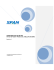### scan0002550 spec sheet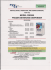### Concrete Form Design - Home - KSU Faculty Member websites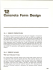### Solutions Manual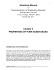### CREEP School 1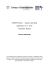### Bubblegum Bump Game +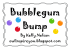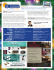### Photo Contest Rules - The Great Forest Park Balloon Race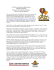### information exchange policy vocabulary (iepv)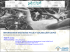### March 2014 - South Central USD 5